# Automate Ground Truth Labeling for OCR

This example shows how to create an automation algorithm to automatically label data for OCR training and evaluation in the Image Labeler app.

### Overview

The Image Labeler, Video Labeler, and Ground Truth Labeler (Automated Driving Toolbox) (Automated Driving Toolbox) apps provide an easy way to interactively label data for training or evaluating image classifiers, object detectors, OCR models, semantic, and instance segmentation networks. These apps include several built-in automation algorithms and an interface to define custom automation algorithms to accelerate the labeling process.

In this example, a custom automation algorithm is created in the Image Labeler app to automatically detect the text regions in images and recognize the words in the detected text regions using a pretrained OCR model.

### Create a Text Detection Algorithm

As described in Train Custom OCR Model, ground truth for OCR consists of the image text location specified as bounding boxes and the actual text content in those locations. The first step in automation is to create a text detection algorithm. This example uses the algorithm described in the Automatically Detect and Recognize Text Using MSER and OCR example to illustrate how to create an automation algorithm.

#### Detect text regions

Load the test image containing text.

```I = imread("DSEG14.jpg"); imshow(I)```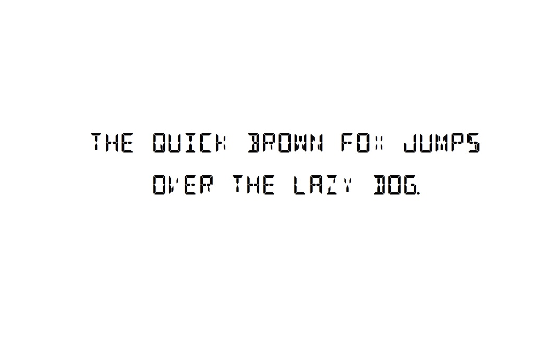`The` `helperDetectTextRegions` function uses techniques described in the Automatically Detect and Recognize Text Using MSER and OCR example to detect candidate text regions. It uses geometric properties of text regions, such as area and aspect ratio, to identify regions that are likely to contain text. For more information, see Automatically Detect and Recognize Text Using MSER and OCR.

Define geometric property thresholds for the helper function. These thresholds may need to be tuned for other images.

```params.MinArea = 20; params.MinAspectRatio = 0.062; params.MaxAspectRatio = 4;```

Use the `helperDetectTextRegions` function to detect text regions in this image.

`bboxes = helperDetectTextRegions(I, params);`

Display text detection results.

`showShape("rectangle",bboxes);`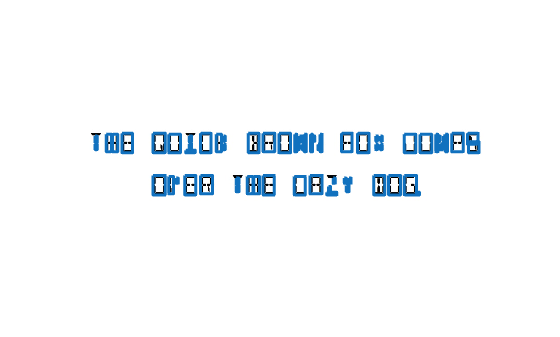#### Detect Word Bounding Boxes

The detected text regions from the previous step must be combined to produce meaningful bounding boxes around words.

Merge the character bounding boxes into word bounding boxes using a distance threshold between characters.

```% Find pairwise distances between bounding boxes. distanceMatrix = helperBboxPairwiseDistance(bboxes); % Define the distance threshold. This threshold may need to be tuned for % other images. maxWordSpacing = 20; % Filter bounding boxes based on distance threshold. connectivity = distanceMatrix < maxWordSpacing; g = graph(connectivity, 'OmitSelfLoops'); componentIndices = conncomp(g); % Merge bounding boxes. bboxes = helperBboxMerge(bboxes, componentIndices'); % Display results. imshow(I); showShape("rectangle", bboxes);```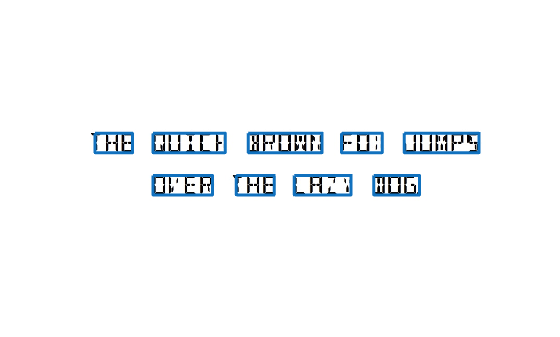The character bounding boxes have been successfully merged into word bounding boxes. Some of the bounding boxes are tightly fit touching the characters. Expand the bounding boxes by 15% so that they do not touch the character. Tune this expansion scale factor for other images such that the bounding boxes do not touch any characters.

```expansionScale = 1.15; bboxes = helperBboxExpand(bboxes, expansionScale);```

Display the resized bounding boxes.

`showShape("rectangle", bboxes);`### Recognize Text using a Pretrained OCR Model

Once the text is detected, you can automatically recognize the text using a pretrained OCR model. In this example, a pretrained OCR model is provided in fourteen-segment.traineddata. Use this model in the `ocr` function to recognize the detected text.

```model = "fourteen-segment.traineddata"; results = ocr(I, bboxes, Model=model , LayoutAnalysis="word");```

Display recognition results.

```imshow(I); showShape("rectangle", bboxes, Label={results.Text}, LabelTextColor="white");```Note that the pretrained OCR model may not provide accurate ground truth labeling. For example, the word QUICK has been incorrectly recognized by the pretrained model. This inaccuracy can be corrected during manual verification after running the automation algorithm by editing the algorithm results.

### Integrate Text Detection Algorithm Into Image Labeler

Incorporate the text detector in the Image Labeler app by creating an automation class in MATLAB that inherits from the abstract base class `vision.labeler.AutomationAlgorithm`. This base class defines the API that the app uses to configure and run the algorithm. The Image Labeler app provides a convenient way to obtain an initial automation class template. The `WordDetectorAutomationAlgorithm` class is based on this template and provides a ready-to-use automation class for text detection.

In this section, some of the key properties and methods of the Automation class are discussed.

The properties section of the automation class specifies the custom properties needed to run the algorithm.

```properties % Properties related to thresholds for word detection. MinArea = 5; MinAspectRatio = 0.062; MaxAspectRatio = 4; MaxWordSpacing = 10; % Properties related to OCR. DoRecognizeText = false; AttributeName = ""; ModelName = "English"; UseCustomModel = false; CustomModel = ""; DoCustomizeCharacterSet = false; CharacterSet = ""; % Properties to cache attributes in the label definition. AttributeList = []; ValidAttributeList = []; end ```

The function, `checkLabelDefinition`, ensures that only labels of the appropriate type are enabled for automation. For OCR labeling, verify that only labels of type Rectangle are enabled and cache any attributes associated with the label definitions.

```function isValid = checkLabelDefinition(this, labelDef) % Only labels for rectangular ROI's are considered valid. isValid = labelDef.Type == labelType.Rectangle; hasAttributes = isfield(labelDef, 'Attributes'); % Cache the attribute list associated with the label definitions. if isValid && hasAttributes attributeNames = fieldnames(labelDef.Attributes); numAttributes = numel(attributeNames); isStringAttribute = false(numAttributes,1); for i = 1:numAttributes if isfield(labelDef.Attributes.(attributeNames{i}), 'DefaultValue') isStringAttribute(i) = ... isstring(labelDef.Attributes.(attributeNames{i}).DefaultValue); end end this.AttributeList = attributeNames; this.ValidAttributeList = attributeNames(isStringAttribute); end end ```

The function, `settingsDialog`, obtains and modifies the properties defined above. Use this API call to create a dialog box that opens when a user clicks the Settings button in the Automate tab. The function uses `helperCreateUIComponents` to create the UI elements in the settings dialog and `helperAttachCallbacks` to attach action callbacks to these created UI elements. Review these functions in the `WordDetectorAutomationAlgorithm`` `class file.

```function settingsDialog(this) app = helperCreateUIComponents(this); helperAttachCallbacks(this, app); end ```

The function, `run,` defines the core algorithms discussed previously in this example. `run` gets called for each image, and expects the automation class to return a set of labels. The `helperDetectWords` function implements the logic discussed in Create a Text Detection Algorithm section. The `helperRecognizeText` implements the logic discussed in Recognize Text using a Pretrained OCR Model section. Review these functions in the `WordDetectorAutomationAlgorithm`` `class file.

```function autoLabels = run(this, I) bboxes = helperDetectWords(this, I); autoLabels = []; if ~isempty(bboxes) autoLabels = helperRecognizeText(this, I, bboxes); end end ```

### Use the Text Detection Automation Class in the App

The properties and methods described in the previous section have been implemented in the WordDetectorAutomationAlgorithm class file. To use this class in the app:

• Create the folder structure `+vision/+labeler` under the current folder, and copy the automation class into it.

```mkdir('+vision/+labeler'); copyfile('WordDetectorAutomationAlgorithm.m','+vision/+labeler'); ```
• Open the Image Labeler app. For illustration purposes, open the CVT-DSEG14.jpg image.• Define a rectangle ROI label and give it a name, for example, 'Text'.

• Define a string attribute for the label and give it a name, for example, 'Word'. The attribute holds the text information for the ROI.

• Click Algorithm > Word Detector. If you do not see this option, ensure that the current working folder has a folder called +vision/+labeler, with a file named WordDetectorAutomationAlgorithm.m in it.• Click Automate. A new panel will open, displaying directions for using the algorithm.

• Click Run. The automated algorithm executes on the image, detecting words. After the run is completed, verify the result of the automation algorithm.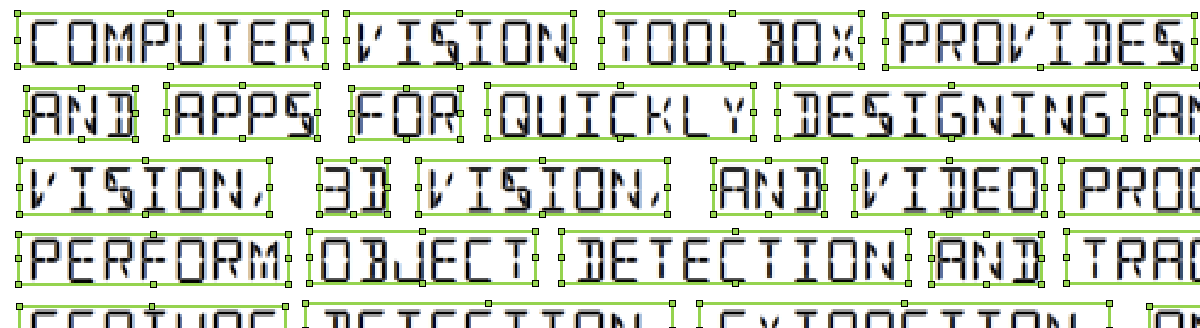• If you are not satisfied with the labels, click Settings. A new dialog will open to display the detection algorithm parameters. Adjust these parameters and rerun the automation algorithm until you get satisfactory results.• In settings dialog, click the Recognize detected words using OCR checkbox to enable Recognition options. The attribute name will populate all the string attributes available for the selected label defintion. Choose Word attribute and select a custom OCR model. Click the Browse button and select the fourteen-segment.traineddata OCR model to recognize the text inside the bounding boxes. Click OK and re-run the automation algorithm.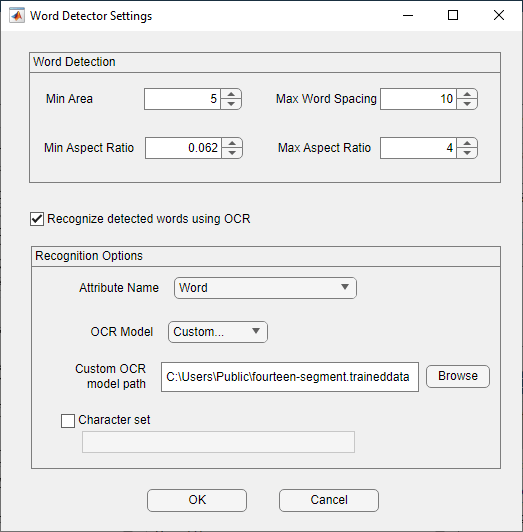• In addition to the detected bounding boxes, the text in them will be recognized and populated in their attribute fields. These can be seen in the View Labels, Sublabels and Attributes section in the right side of the App.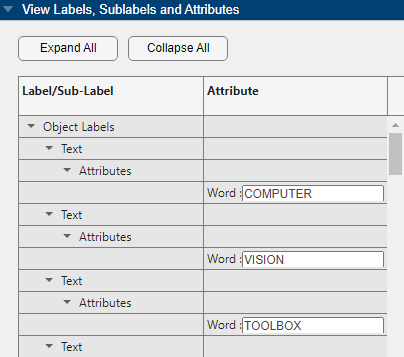• Automation for OCR labeling for the image is now complete. Manually verify the text bounding boxes and the recognized text in the attribute fields.

• Click Accept to save and export the results of this labeling run.

### Conclusion

This example demonstrated how to detect words in images using geometric properties of text and recognize them using a pretrained OCR model to accelerate labeling of text in Image Labeler app using the `AutomationAlgorithm` interface. If a text detector based on geometric properties is not sufficient, use the steps described in this example to create an automation algorithm that uses a pretrained text detector based on deep learning. For more information, see `detectTextCRAFT` and Automatically Detect and Recognize Text Using Pretrained CRAFT Network and OCR.

### Supporting Functions

#### `helperDetectTextRegions` function

The `helperDetectTextRegions` function detects bounding boxes around connected components in the image and filters them using geometric properties such as area, aspect ratio and overlap.

```function bboxes = helperDetectTextRegions(in, params) % Binarize the image. bw = helperBinarizeImage(in); % Find candidate bounding boxes for text regions. cc = bwconncomp(bw); stats = regionprops(cc, {'BoundingBox'}); bboxes = vertcat(stats(:).BoundingBox); % Filter bounding boxes based on minimum area. area = prod(bboxes(:,[3 4]), 2); toRemove = area < params.MinArea; % Filter bounding boxes based on minimum and maxium aspect ratio. aspectRatio = bboxes(:,3)./bboxes(:,4); toRemove = toRemove | (aspectRatio < params.MinAspectRatio | aspectRatio > params.MaxAspectRatio); % Filter bounding boxes based on overlap ratio. overlap = bboxOverlapRatio(bboxes, bboxes, 'min'); % remove boxes that overlap more than 5 other boxes overlap(toRemove,:) = 0; % do not count those boxes that are to be removed. numChildren = sum(overlap > 0) - 1; % -1 for self toRemove = toRemove | numChildren' > 5; % Remove filtered bounding boxes. bboxes(toRemove, :) = []; % Find overlapping bounding boxes. overlap = bboxOverlapRatio(bboxes,bboxes, 'min'); g = graph(overlap > 0.5, 'OmitSelfLoops'); componentIndices = conncomp(g); % Merge bounding boxes. bboxes = helperBboxMerge(bboxes, componentIndices'); end```

#### `helperBinarizeImage` function

The `helperBinarizeImage` function binarizes the image and inverts the binary image if the text in the image is darker than the background.

```function I = helperBinarizeImage(I) if ~ismatrix(I) I = rgb2gray(I); end if ~islogical(I) I = imbinarize(I); end % determine text polarity; dark on light vs. light on dark. % For text detection, we want light on dark. c = imhist(I); [~,bin] = max(c); if bin == 2 % light background % complement image to switch polarity I = imcomplement(I); end end```

#### `helperBboxMerge` function

The `helperBboxMerge` function merges bounding boxes based on group indices. `inBboxes` is a M-by-4 vector and `outBboxes` is a N-by-4 vectors. `groupIndices` is a M-by-1 label vector corresponding to its merge group (1, ... ,N).

```function outBboxes = helperBboxMerge(inBboxes, groupIndices) % Convert the [x y width height] coordinates to start and end coordinates. xmin = inBboxes(:,1); ymin = inBboxes(:,2); xmax = xmin + inBboxes(:,3) - 1; ymax = ymin + inBboxes(:,4) - 1; % Merge the boxes based on the minimum and maximum dimensions. xmin = accumarray(groupIndices, xmin, [], @min); ymin = accumarray(groupIndices, ymin, [], @min); xmax = accumarray(groupIndices, xmax, [], @max); ymax = accumarray(groupIndices, ymax, [], @max); outBboxes = [xmin ymin xmax-xmin+1 ymax-ymin+1]; end```

#### `helperBboxPairwiseDistance` function

The `helperBboxPairwiseDistance` function computes pairwise distances between bounding boxes. The distance between two bounding boxes is defined as the distance between their closest edges. `bboxes` is a M-by-4 vector of bounding boxes. `dists` is a M-by-M matrix of pairwise distances.

```function dists = helperBboxPairwiseDistance(bboxes) numBoxes = size(bboxes, 1); dists = zeros(numBoxes); % Populate distance matrix row by row by computing distance between one % bounding box to all other bounding boxes iteratively. for i = 1:numBoxes % Pick a bounding box to start with. bbox1 = bboxes(i,:); % Convert bounding boxes to corner points. point1 = bbox2points(bbox1); points = bbox2points(bboxes); % Find centroid of the bounding boxes. centroid1 = permute(mean(point1), [3 2 1]); centroids = permute(mean(points), [3 2 1]); % Compute distance between their closest edges. w1 = bbox1(3); h1 = bbox1(4); ws = bboxes(:,3); hs = bboxes(:,4); xDists = abs(centroid1(1)-centroids(:,1)) - (w1+ws)/2; yDists = abs(centroid1(2)-centroids(:,2)) - (h1+hs)/2; dists1 = max(xDists, yDists); dists1(dists1 < 0) = 0; % Store the result in the distance matrix. dists(:, i) = dists1; end end```

#### `helperBboxExpand` function

The `helperBboxExpand` function returns a bounding box `bboxOut` that is `scale` times the size of `bboxIn`. `bboxIn` and `bboxOut` are M-by-4 vectors of input and output bounding boxes respectively. `scale` is a scalar specifying the resize factor.

```function bboxOut = helperBboxExpand(bboxIn, scale) % Convert input bounding boxes to corner points. points = bbox2points(bboxIn); % Find centroid of the input bounding boxes. centroids = permute(mean(points), [3 2 1]); % Compute width and height of output bounding boxes. newWidth = scale*bboxIn(:,3); newHeight = scale*bboxIn(:,4); % Find the coordinates of the output bounding boxes. newX = centroids(:,1) - newWidth/2; newY = centroids(:,2) - newHeight/2; bboxOut = [newX, newY, newWidth, newHeight]; end```